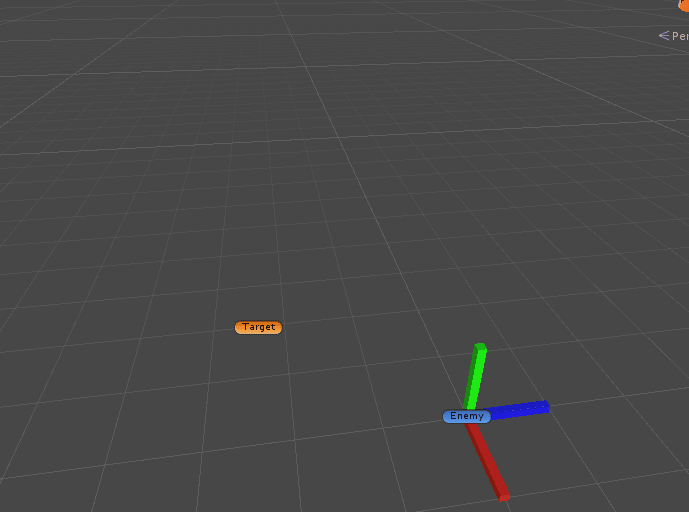2 views

The problem is that I cannot make a script that makes the enemy rotate so that "up" is pointing against the player, (with that I mean so that it will come to the player with transform.up) And I have not found any working solution yet, (from what I can tell)

I am trying to make a small 2D game, just for my friends to play. What I have tried is making the enemy look at the player and then set the "z" rotation to the same as the "x" rotation and after that reset the "x" and "y" rotation (so that "up" is pointing at the player). But the enemy just goes straight up.

public class Enemy : MonoBehaviour

{

public Transform Player;  // The Transform of the Player that you can controll

public float MoveSpeed = 4; // How fast the enemy should go

void FixedUpdate()

{

transform.LookAt(Player);

transform.rotation = Quaternion.Euler(transform.rotation.x, 0, transform.rotation.x);

transform.rotation = Quaternion.Euler(0, 0, transform.rotation.z);

transform.position += transform.up * MoveSpeed * Time.deltaTime;

}

}

So what I want to happen is that the Enemy will move to the Player but the rotation part is not working and it just goes straight up in the air instead of following the player. I might just be stupid and have missed something simple...

by (108k points)

The easiest way I can think of is to make an empty GameObject, that will be the parent of your mesh.

Then, rotate the child (your mesh), so that the part you refer to as "UP", is in-line with the parent's transform.up(positive y-axis).

Once you've done that, you can simply use transform.LookAt(character), but using the parent object's transform.

It depends a bit on how exactly you want the enemy rotated in the end but you could simply use Transform.RotateAround like

• transform.LookAt(Player);

• transform.RotateAround(transform.position, transform.right, 90);

• LookAt by default results in rotation such that transform.forward is pointing at the target, transform.right remains pointing right(ish) and transform.up remains pointing up(ish). So all you have to do is rotating around that local X-axis transform. right exactly 90° so now transform.forward points down instead and transform.up points at the target.

• which results in

•• If you then also want to change the other axis you can still additionally rotate it around the local transform. up axis as well e.g. to flip the forward vector "upwards":

• transform.LookAt(Player);

• transform.RotateAround(transform.position, transform.right, 90);

• transform.RotateAround(transform.position, transform.up, 180);